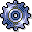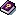Quantity DeterminationUse

Quantity determination uses formulas to calculate the object amounts used in the evaluation of a template. You can, for example, use the R/3 System to calculate the quantity of a sender object (for example, a business process) utilized by a cost object.

You define the formulas for the quantity calculation in the editor.Example: Quantity Determination (Template for cost object/costing)

Example: Quantity Determination (Template for formula planning/cost centers)